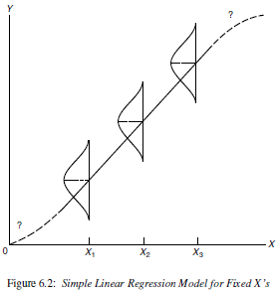## 7.2 Mathematical Model

The mathematical model that we use for regression has three features.

1. $$Y$$ values are normally distributed at any given $$X$$
2. The mean of $$Y$$ values at any given $$X$$ follows a straight line $$Y = \beta_{0} + \beta_{1} X$$.
3. The variance of $$Y$$ values at any $$X$$ is $$\sigma^2$$ (same for all X). This is known as homoscedasticity, or homogeneity of variance.

Mathematically this is written as:

$Y|X \sim N(\mu_{Y|X}, \sigma^{2}) \\ \mu_{Y|X} = \beta_{0} + \beta_{1} X \\ Var(Y|X) = \sigma^{2}$

and can be visualized as:Figure 6.2

### 7.2.1 Unifying model framework

The mathematical model above describes the theoretical relationship between $$Y$$ and $$X$$. So in our unifying model framework to describe observed data,

DATA = MODEL + RESIDUAL

Our observed data values $$y_{i}$$ can be modeled as being centered on $$\mu_{Y|X}$$, with normally distributed residuals.

$y_{i} = \beta_{0} + \beta_{1} X + \epsilon_{i} \\ \epsilon_{i} \sim N(0, \sigma^{2})$